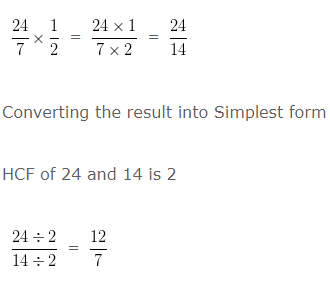# Multiplying Fractions

### Multiplying Fractions by Whole Numbers:

• When any fractions either proper or improper are multiplied with a whole number, the numerators are multiplied with whole number and the denominator will remains the same.
• In generalise form, fraction$\cfrac{a}{b}$ and whole number ‘c’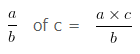Example

Find:

3/12 of 6

Explanation

In this question ‘of’ means to multiply

When any fractions either proper or improper are multiplied with a whole number, the numerators are multiplied with whole number and the denominator will remains the same.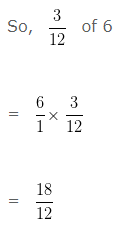HCF of 18 and 12 is 6

Since, both numerator and denominator are divide by their HCF i.e,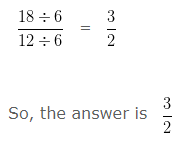### Multiplying Fractions by Fractions:

• When any fractions either proper or improper are multiplied, the numerators are multiplied with each other and the denominators are multiplied with each other.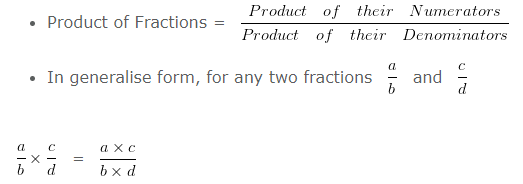Example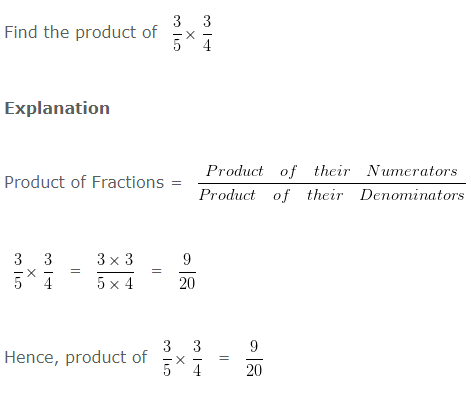### Multiplying Mixed Fractions with Fractions

• When a mixed fraction is multiplied by a proper or improper fraction, convert mixed fraction into improper fraction and then multiply them.

Example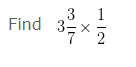Explanation

In order to multiply a Mixed Fraction with a Improper/ Proper Fraction, we first convert mixed Fraction into Improper Fraction , and then multiply it with the Improper/Proper Fraction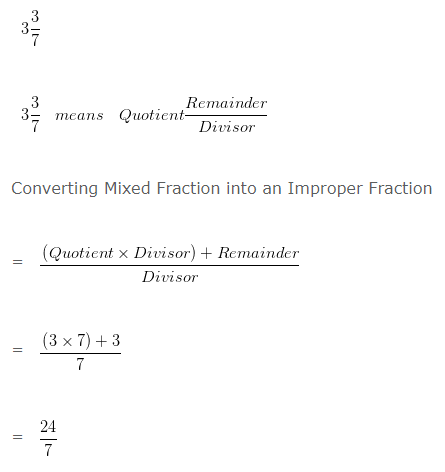Multiplying the Improper Fraction obtained by conversion of Mixed Fraction, with given Fraction